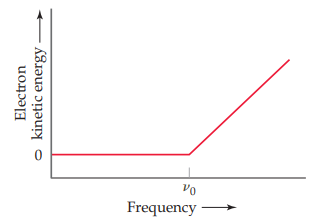×
Get Full Access to Chemistry: The Central Science - 12 Edition - Chapter 6 - Problem 83ae
Get Full Access to Chemistry: The Central Science - 12 Edition - Chapter 6 - Problem 83ae

×

# In an experiment to study the photoelectric effect, aISBN: 9780321696724 27

## Solution for problem 83AE Chapter 6

Chemistry: The Central Science | 12th Edition

• Textbook Solutions
• 2901 Step-by-step solutions solved by professors and subject experts
• Get 24/7 help from StudySoup virtual teaching assistantsChemistry: The Central Science | 12th Edition

4 5 1 329 Reviews
18
5
Problem 83AE

In an experiment to study the photoelectric effect, a scientist measures the kinetic energy of ejected electrons as a function of the frequency of radiation hitting a metal surface. She obtains the following plot. The point labeled "" corresponds to light with a wavelength of. (a) What is the value ofinWhat is the value of the work function of the metal in units ofof ejected electrons? (c) What happens when the metal is irradiated with light of frequency less than(d) Note that when the frequency of the light is greater than, the plot shows a straight line with a nonzero slope. Why is this the case? (e) Can you determine the slope of the line segment discussed in part (d)? Explain.Step-by-Step Solution:
Step 1 of 3

Note taker: Sera ASTR 1345 Light and Energy Observational Astronomers  Use telescopes to study actual events and phenomena  Ex. Edwin Hubble, Galileo Galilei Theoretical Astronomers  Use mathematics and physics-based predictions and theories  Ex. Stephen Hawking, Kip Thorne Computational Astronomers  Recreate and test complex physical situations with computers  Ex. Roadrunner Computer (1,700,000,000,000,000 calculations per second) Where Light Comes From  Emitted: an object gives off energy o Depends strongly on temperature  Reflected o Emitted light from one object bounces off of another  Scattered o Chemicals reflect light unevenly White Light  Newton’s Prism o White light (like sunlight) is a combination of all visible colors o A medium of different density will cause light to reflect (bend) Refraction  When light is passed through a medium, the path of it gets deviated  Light only travels in a straight line at the speed of light “The Light Bucket” - The Telescope  A simple refracting telescope o Uses lenses to refract light o Most important astronomical invention  Human vision is unreliable o Light enters the small pupil and is focused light detecting cells

Step 2 of 3

Step 3 of 3

## Discover and learn what students are asking

Statistics: Informed Decisions Using Data : Inference about Two Population Standard Deviations
?In Problems 9–14, assume that the populations are normally distributed. Test the given hypothesis. 12. s1 6 s2 at the a = 0.05 level of signi

Unlock Textbook Solution